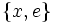# 2-subnormal not implies automorph-permutable

This article gives the statement and possibly, proof, of a non-implication relation between two subgroup properties. That is, it states that every subgroup satisfying the first subgroup property (i.e., 2-subnormal subgroup) need not satisfy the second subgroup property (i.e., automorph-permutable subgroup)
View a complete list of subgroup property non-implications | View a complete list of subgroup property implications
EXPLORE EXAMPLES YOURSELF: View examples of subgroups satisfying property 2-subnormal subgroup but not automorph-permutable subgroup|View examples of subgroups satisfying property 2-subnormal subgroup and automorph-permutable subgroup

## Statement

### Property-theoretic statement

The subgroup property of being a 2-subnormal subgroup is not stronger than the subgroup property of being an automorph-permutable subgroup.

### Verbal statement

A 2-subnormal subgroup (a subgroup that is normal in a normal subgroup) need not be automorph-permutable.

## Proof

### Example of a dihedral group of order eight

Consider the dihedral group of order eight, generated by a rotation$a$ and a reflection$x$. Then, the two-element subgroup generated by$x$ is a 2-subnormal subgroup (it is not normal, but its normal closure is$\{ e, a^2, x, a^2x \}$, which is normal).

On the other hand, the subgroup$\{ x , e \}$ does not permute with the subgroup$\{ ax , e \}$, which is its image under a suitable outer automorphism.